# NCERT Solutions For Class 11 Physics Motion in A Straight Line## myCBSEguide App

CBSE, NCERT, JEE Main, NEET-UG, NDA, Exam Papers, Question Bank, NCERT Solutions, Exemplars, Revision Notes, Free Videos, MCQ Tests & more.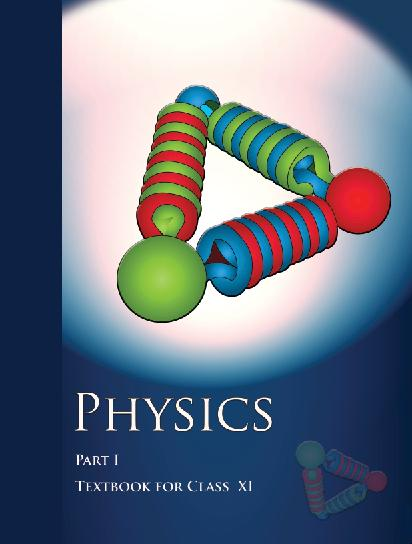## NCERT Class 11 Physics Chapter wise Solutions

• 1 – Physical World
• 2 – Units and Measurements
• 3 – Motion in a Straight line
• 4 – Motion in a Plane
• 5 – Laws of Motion
• 6 – Work, Energy and Power
• 7 – System of Particles and Rotational motion
• 8 – Gravitation
• 9 – Mechanical Properties of Solids
• 10 – Mechanical properties of fluids
• 11 – Thermal Properties of matter
• 12 – Thermodynamics
• 13 – Kinetic Theory
• 14 – Oscillations
• 15 – Waves

### CHAPTER 3 MOTION IN A STRAIGHT LINE

• 3.1 Introduction
• 3.2 Position, pathy length and displacement
• 3.3 Average velocity and average speed
• 3.4 Instantaneous velocity and speed
• 3.5 Acceleration
• 3.6 Kinematic equations for uniformly accelerated motion
• 3.7 Relative velocity

## NCERT Solutions For Class 11 Physics Motion in A Straight Line

1. In which of the following examples of motion, can the body be considered approximately a point object:

(a) a railway carriage moving without jerks between two stations.

(b) a monkey sitting on top of a man cycling smoothly on a circular track.

(c) a spinning cricket ball that turns sharply on hitting the ground.

(d) a tumbling beaker that has slipped off the edge of a table.

2. The position-time (x-t) graphs for two children A and B returning from their school O to their homes P and Q respectively are shown in Fig. 3.19. Choose the correct entries in the brackets below;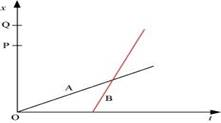(a) (A/B) lives closer to the school than (B/A)

(b) (A/B) starts from the school earlier than (B/A)

(c) (A/B) walks faster than (B/A)

(d) A and B reach home at the (same/different) time

(e) (A/B) overtakes (B/A) on the road (once/twice).

3. A woman starts from her home at 9.00 am, walks with a speed of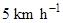on a straight road up to her office 2.5 km away, stays at the office up to 5.00 pm, and returns home by an auto with a speed of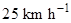. Choose suitable scales and plot the xt graph of her motion.

4. A drunkard walking in a narrow lane takes 5 steps forward and 3 steps backward, followed again by 5 steps forward and 3 steps backward, and so on. Each step is 1 m long and requires 1 s. Plot the xt graph of his motion. Determine graphically and otherwise how long the drunkard takes to fall in a pit 13 m away from the start.

5. A jet airplane travelling at the speed of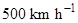ejects its products of combustion at the speed of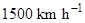relative to the jet plane. What is the speed of the latter with respect to an observer on ground?

6. A car moving along a straight highway with a speed of 126km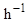is brought to a stop within a distance of 200m. What is the retardation of the car (assumed uniform), and how long does it take for the car to stop?

7. Two trains A and B of length 400 m each are moving on two parallel tracks with a uniform speed of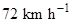in the same direction, with A ahead of B. The driver of B decides to overtake A and accelerates by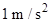. If after 50 s, the guard of B just brushes past the driver of A, what was the original distance between them?

8. On a two-lane road, car A is travelling with a speed of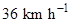. Two cars B and C approach car A in opposite directions with a speed of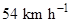each. At a certain instant, when the distance AB is equal to AC, both being 1 km, B decides to overtake A before C does. What minimum acceleration of car B is required to avoid an accident?

9. Two towns A and B are connected by a regular bus service with a bus leaving in either direction every T minutes. A man cycling with a speed of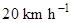in the direction A to B notices that a bus goes past him every 18 min in the direction of his motion, and every 6 min in the opposite direction. What is the period T of the bus service and with what speed (assumed constant) do the buses ply on the road?

10. A player throws a ball upwards with an initial speed of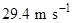.

(a) What is the direction of acceleration during the upward motion of the ball?

(b) What are the velocity and acceleration of the ball at the highest point of its motion?

(c) Choose the x = 0 m and t = 0 s to be the location and time of the ball at its highest point, vertically downward direction to be the positive direction of x-axis, and give the signs of position, velocity and acceleration of the ball during its upward, and downward motion.

(d) To what height does the ball rise and after how long does the ball return to the player’s hands? (Take g = 9.8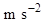and neglect air resistance).

11. Read each statement below carefully and state with reasons and examples, if it is true or false;

A particle in one-dimensional motion

(a) with zero speed at an instant may have non-zero acceleration at that instant

(b) with zero speed may have non-zero velocity,

(c) with constant speed must have zero acceleration,

(d) with positive value of acceleration must be speeding up.

12. A ball is dropped from a height of 90 m on a floor. At each collision with the floor, the ball loses one tenth of its speed. Plot the speed-time graph of its motion between t = 0 to 12 s.

13. Explain clearly, with examples, the distinction between:

(a) magnitude of displacement (sometimes called distance) over an interval of time, and the total length of path covered by a particle over the same interval;

(b) magnitude of average velocity over an interval of time, and the average speed over the same interval. [Average speed of a particle over an interval of time is defined as the total path length divided by the time interval]. Show in both (a) and (b) that the second quantity is either greater than or equal to the first.

When is the equality sign true? [For simplicity, consider one-dimensional motion only].

14. A man walks on a straight road from his home to a market 2.5 km away with a speed of 5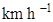. Finding the market closed, he instantly turns and walks back home with a speed of 7.5 km h-1. What is the

(a) magnitude of average velocity, and

(b) average speed of the man over the interval of time (i) 0 to 30 min, (ii) 0 to 50 min, (iii) 0 to 40 min? [Note: You will appreciate from this exercise why it is better to define average speed as total path length divided by time, and not as magnitude of average velocity. You would not like to tell the tired man on his return home that his averages speed was zero!]

15. In Exercises 3.13 and 3.14, we have carefully distinguished between average speed and magnitude of average velocity. No such distinction is necessary when we consider instantaneous speed and magnitude of velocity. The instantaneous speed is always equal to the magnitude of instantaneous velocity. Why?

16. Look at the graphs (a) to (d) (Fig. 3.20) carefully and state, with reasons, which of these cannot possibly represent one-dimensional motion of a particle.

(a)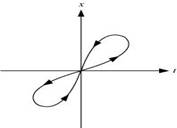(b)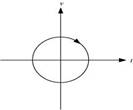(c)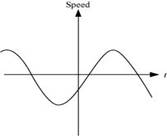(d)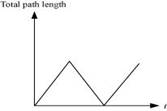17. Figure 3.21 shows the x-t plot of one-dimensional motion of a particle. Is it correct to say from the graph that the particle moves in a straight line for t < 0 and on a parabolic path for t > 0? If not, suggest a suitable physical context for this graph.

18. A police van moving on a highway with a speed of 30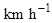fires a bullet at a thief’s car speeding away in the same direction with a speed of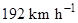. If the muzzle speed of the bullet is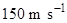, with what speed does the bullet hit the thief’s car ? (Note: Obtain that speed which is relevant for damaging the thief’s car).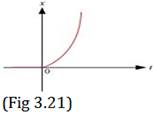19. Suggest a suitable physical situation for each of the following graphs (Fig 3.22):

(a)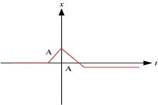(b)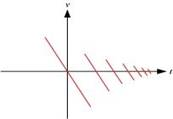(c)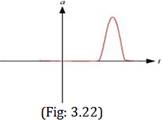20. Figure 3.23 gives the x-t plot of a particle executing one-dimensional simple harmonic motion. (You will learn about this motion in more detail in Chapter14). Give the signs of position, velocity and acceleration variables of the particle at t = 0.3 s, 1.2 s, – 1.2 s.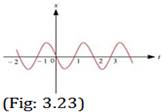21. Figure 3.24 gives the x-t plot of a particle in one-dimensional motion. Three different equal intervals of time are shown. In which interval is the average speed greatest, and in which is it the least? Give the sign of average velocity for each interval.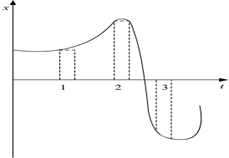(Fig: 3.24)

22. Figure 3.25 gives a speed-time graph of a particle in motion along a constant direction. Three equal intervals of time are shown. In which interval is the average acceleration greatest in magnitude? In which interval is the average speed greatest? Choosing the positive direction as the constant direction of motion, give the signs of v and a in the three intervals. What are the accelerations at the points A, B, C and D?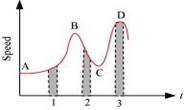(Fig: 3.25)23. A three-wheeler starts from rest, accelerates uniformly with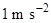on a straight road for 10 s, and then moves with uniform velocity. Plot the distance covered by the vehicle during the nth second (n = 1,2,3….) versus n. What do you expect this plot to be during accelerated motion: a straight line or a parabola?

24. A boy standing on a stationary lift (open from above) throws a ball upwards with the maximum initial speed he can, equal to 49 m/s. How much time does the ball take to return to his hands? If the lift starts moving up with a uniform speed of 5 m/s and the boy again throws the ball up with the maximum speed he can, how long does the ball take to return to his hands?

25. On a long horizontally moving belt (Fig. 3.26), a child runs to and fro with a speed 9 km h-1 (with respect to the belt) between his father and mother located 50 m apart on the moving belt. The belt moves with a speed of 4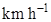. For an observer on a stationary platform outside, what is the

(a) speed of the child running in the direction of motion of the belt ?.

(b) speed of the child running opposite to the direction of motion of the belt ?

(c) time taken by the child in (a) and (b) ?

Which of the answers alter if motion is viewed by one of the parents?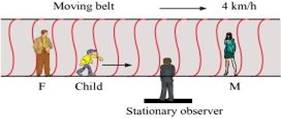(Fig: 3.26)

26. Two stones are thrown up simultaneously from the edge of a cliff 200 m high with initial speeds of 15 m/s and 30 m/s. Verify that the graph shown in Fig. 3.27 correctly represents the time variation of the relative position of the second stone with respect to the first. Neglect air resistance and assume that the stones do not rebound after hitting the ground. Take g =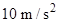. Give the equations for the linear and curved parts of the plot.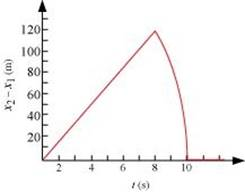27. The speed-time graph of a particle moving along a fixed direction is shown in Fig. 3.28. Obtain the distance traversed by the particle between (a) t = 0 s to 10 s, (b) t = 2 s to 6 s.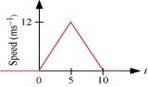(Fig. 3.28)

What is the average speed of the particle over the intervals in (a) and (b)?

28. The velocity-time graph of a particle in one-dimensional motion is shown in Fig. 3.29: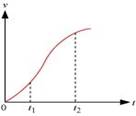Which of the following formulae are correct for describing the motion of the particle over the time-interval t2 to t1?

(a)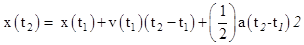(b)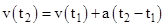(c) vAverage =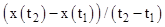(d) aAverage =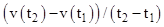(e)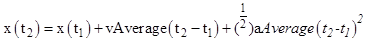(f)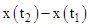= area under the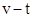curve bounded by the t-axis and the dotted line shown.

## NCERT Solutions for Class 11 Physics

NCERT Solutions Class 11 Physics PDF (Download) Free from myCBSEguide app and myCBSEguide website. Ncert solution class 11 physics includes text book solutions from both part 1 and part 2. NCERT Solutions for CBSE Class 11 Physics have total 15 chapters. 11 Physics NCERT Solutions in PDF for free Download on our website. Ncert physics class 11 solutions PDF and physics ncert class 11 PDF solutions with latest modifications and as per the latest CBSE syllabus are only available in myCBSEguide

## CBSE app for Class 11

To download NCERT Solutions for class 11 Physics, Chemistry, Biology, History, Political Science, Economics, Geography, Computer Science, Home Science, Accountancy, Business Studies and Home Science; do check myCBSEguide app or website. myCBSEguide provides sample papers with solution, test papers for chapter-wise practice, NCERT solutions, NCERT Exemplar solutions, quick revision notes for ready reference, CBSE guess papers and CBSE important question papers. Sample Paper all are made available through the best app for CBSE students and myCBSEguide website